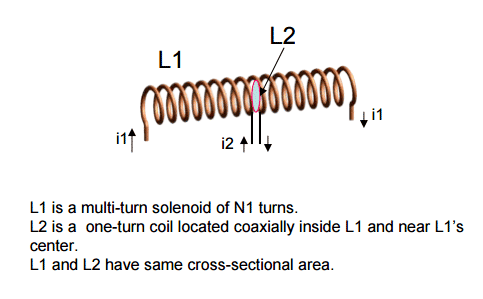# Misconceiving Mutual Inductance Coefficients

[Total: 2    Average: 5/5]A commonly used formula for mutual inductance M between two nearby coils L1 and L2 is M = k√(L1*L2). This formula however assumes equal percentage flux linkages between the two coils. This requirement is often omitted in several of the references I have looked at.

That this requirement is not necessarily met can be seen by looking at fig. 1, which depicts a single-turn coil L2 imbedded within a long solenoid L1 with N1 turns. It is obvious that flux from L1 essentially totally links L2, whereas flux from L2 equally obviously is coupled only partially into L1.

As is well known, M21 = M12 = M. (The proof is not trivial; Feynman uses the magnetic vector potential while Skilling invokes an energy argument.)

The following fallacious reasoning can ensue, and I speak from experience! So M can be computed either way per the above. Obviously, it’s much easier to go with M21 since φ21 = φ11 . I won’t derive the result, which is easily done and is M = μ0A/l in vacuum
where A = cross-sectional area of both coils and l = length of L1.

So now we say OK, there is 100% flux coupling between L1 and L2 so k = 1 and M = the (too!) familiar k√(L1L2). WRONG!

Were it right we could do an amazing thing: we could easily compute L2 which otherwise happens to be an exceedingly difficult task; L2 depends on A and the wire radius R and can only be derived from a special integral to some arbitrary order. But – we know L1, we know M and we think we know k so L2 can easily be computed!

Not so! The result of this fallacious computation would imply that L2 is a function of L1, even in the total absence of solenoid current i1! And you could also ignore little inconvenient realities like L2 → ∞ as R → 0!

The thing is, there are really two coupling coefficients. Define
k1 = φ2111 and
k2 = φ1222. Then we can perform a few substitutions based on the above to find that
M = √(k1k2L1L2).

Bottom line here is that we know k1 but not k2, and L1 but not L2. There is one coupling coefficient k = k1 = k2 if and only if the fraction of flux from one coil coupling into the other is the same for both coils.

Even if the single-turn coil were replaced by another long solenoid L2, but of different length, wrapped on top of L1 so that A is the same for both, and L2 were as easily computed as L1, still there are two different coupling coefficients involved, and the familiar formula L2/L1 = (N2/N1)2 is incorrect.

Tags:
4 replies
1.Lebogang says:

How does a coil work  with matching box in an ion source chamber when producing a beam or making plasma,i am all confused as i am a student?

2.rude man says:

Nice work @rude man

Thank you!

3.rude man says:

How does a coil work with matching box in an ion source chamber when producing a beam or making plasma,i am all confused as i am a student?

I am not sure what you mean by a “matching box” and “making plasma”; could you give me a link that describes this setup?